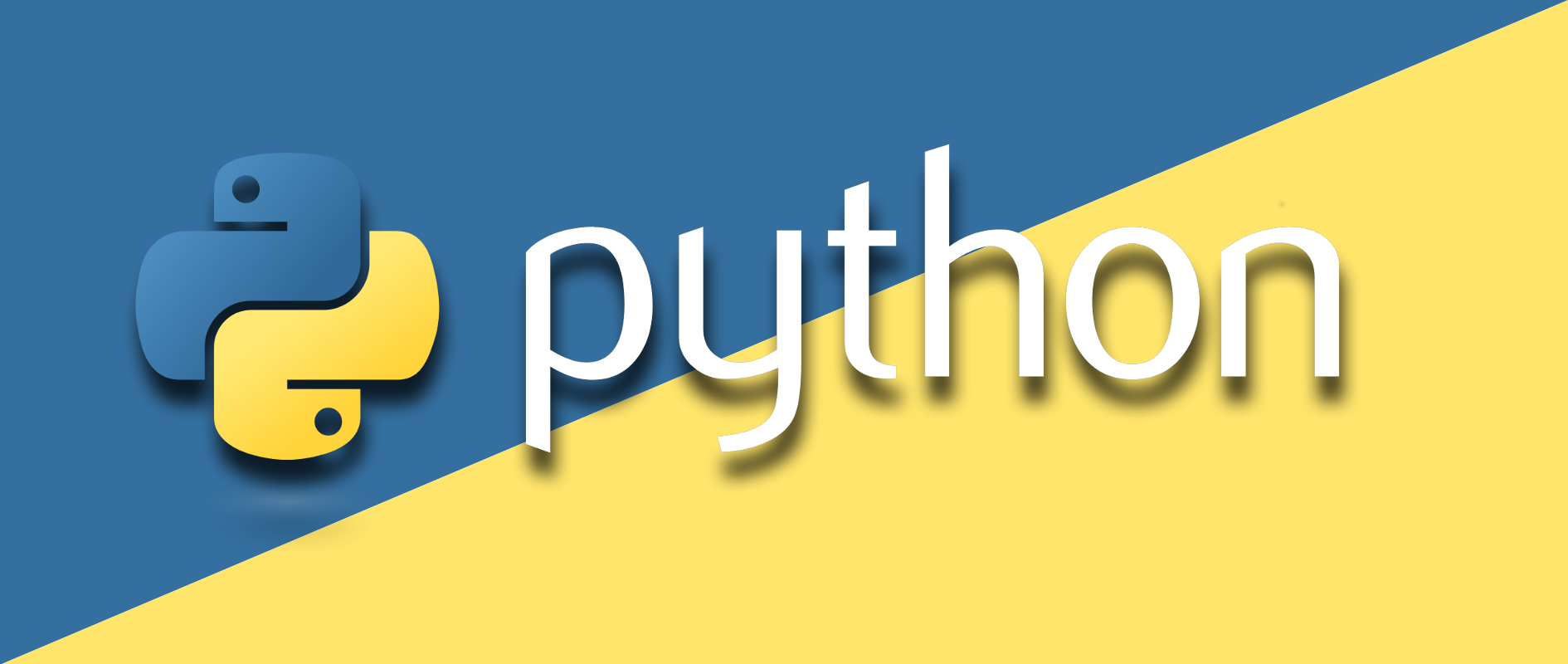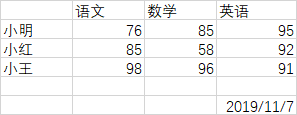# 小白学 Python（23）：Excel 基础操作（上）## 读取 ExcelExcel 内容如下：Excel 自带的函数确实强大，这个不得不服，但是，我如果要跨 Sheet 的操作呢？本文要玩的骚操作就是要跨 Sheet 计算平均成绩。pip install xlrd


workbook = xlrd.open_workbook('test.xlsx')


Sheet 有关操作：

# 输出所有 sheet 的名字
print(workbook.sheet_names())
# 获取所有的 sheet
print(workbook.sheets())
# 根据索引获取 sheet
print(workbook.sheet_by_index(1))
# 根据名字获取 sheet
print(workbook.sheet_by_name('1班'))


sheet1 = workbook.sheets()
# 获取行数
print(sheet1.nrows)
# 获取列数
print(sheet1.ncols)


6
4


# 获取第 2 行内容
print(sheet1.row_values(1))
# 获取第 3 列内容
print(sheet1.col_values(2))


['小明', 76.0, 85.0, 95.0, '']
['数学', 85.0, 58.0, 96.0, '', '']


cell1 = sheet1.cell(1, 1).value
# 行索引
cell2 = sheet1.row(1).value
cell3 = sheet1.cell(1, 2).value
# 列索引
cell4 = sheet1.col(2).value


76.0 76.0 85.0 85.0


date_value = xlrd.xldate_as_datetime(sheet1.cell_value(5, 3), workbook.datemode)
print(type(date_value), date_value)


<class 'datetime.datetime'> 2019-11-07 20:49:05


date_tulp = xlrd.xldate_as_tuple(sheet1.cell_value(5, 3), workbook.datemode)
print(type(date_tulp), date_tulp)
year, month, day, hour, minute, second = date_tulp
print(datetime.datetime(year, month, day, hour, minute, second))


<class 'tuple'> (2019, 11, 7, 20, 49, 5)
2019-11-07 20:49:05## 示例代码

posted @ 2019-11-18 08:44  极客挖掘机  阅读(368)  评论(0编辑  收藏